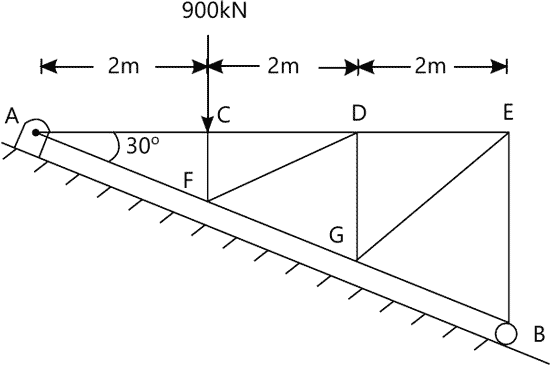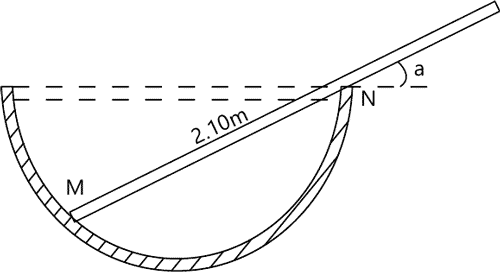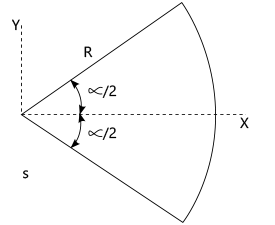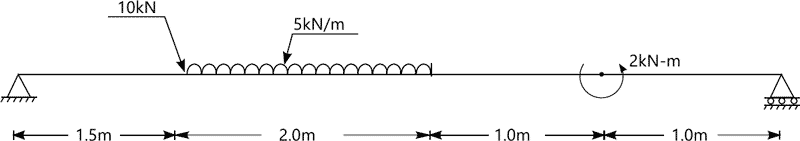MORE IN Basic Civil Engg. & Engg. Mechanics
RGPV First Year Engineering (Set B) (Semester 1)
Basic Civil Engg. & Engg. Mechanics
May 2014
Total marks: --
Total time: --
INSTRUCTIONS
(1) Assume appropriate data and state your reasons
(2) Marks are given to the right of every question
(3) Draw neat diagrams wherever necessary

1 (a) What do you mean by M-30 concrete? Explain.
2 M
1 (b) Enumerate and describe the tests which may be performed in field to assess the quality ofbricks.
2 M
1 (c) What do you mean by workability of the concrete? Explain about the various factors affecting it.
3 M
Answer any one question from Q1.(d) & Q1.(e)
1 (d) What are the objectives for providing foundation in the building? Draw a neat sketch of RCC circular column footing with details of column and pedestal.
7 M
1 (e) Mention the various factors considered while choosing location of stair in a building. Draw neat sketch of Dog-legged stair showing various components of the stair.
7 M

2 (a) What do you mean by transit and non transit theodolite?
2 M
2 (b) What are the various fundamental lines in a theodolites / level and what is the desired relation amongst them?
2 M
2 (c) Prove that the curvature and refraction errors are eliminated in reciprocal leveling.
3 M
Answer any one question from Q2.(d) & Q2.(e)
2 (d) Following consecutive readings were taken on a continuously sloping ground at regular interval of 30m. Find the gradient joining first and last point (using 3m leveling staff) 1.370m, 2.055m, 2.760m, 0.455m, 1.110m, 1.875m, 2.57m, 0.305m, 0.995m, 1.685m. Enter the above readings in a page of field and level book. Deduce RL of intermediate points using H.I. method.
7 M
2 (e) Explain how plotting can be done by plate table surveying using method of traversing. Mention step by step procedure.
7 M

3 (a) Explain the factors on which contour interval depends?
2 M
3 (b) Draw contours of ridge and a valley line assigning suitable values to the contour shown in the figure (in your answer).
2 M
3 (c) Write an explanatory note on applications of remote sensing
3 M
Answer any one question from Q3.(d) & Q3.(e)
3 (d) A Rectangular Plot abcd forms the plane of a pit for borrowing Earth from it eis point of intersection of the diagonals. Calculate Volume of excavation using following data.
 Point a b c s e Original levels(m) 42.2 49.8 51.2 47.2 52.0 Final level(m) 38.6 39.8 42.6 40.8 42.5 Provided Length ab=50m bc=80m
7 M
3 (e) Explain How contour maps can be used to draw cross sectioning and to find out capacity of a reservoir.
7 M

4 (a) State and explain theorem of varignon.
2 M
4 (b) Show how a force acting on a body can be replaced by an equivalent force couple system.
2 M
4 (c) How determinacy and stability of a frame can be ascertained.
3 M
Answer any one question from Q4.(d) & Q4.(e)
4 (d) Determine axial forces in the members CF,FD,DG,GE and EB of Simply supported loaded truss as shown in fig.17 M
4 (e) Prismatic bar of length 2.1m and weight 365N rests with in a hemispherical bowl of radius 0.7m as shown in Fig.2. Determine angle for position of equilibrium7 M

5 (a) What do you mean by polar moment of inertia?
2 M
5 (b) What are the various of types of supports of a beam?
2 M
5 (c) What do you understand by second moment of area and radius of Gyration? Explain.
3 M
Answer any one question from Q5.(d) & Q5.(e)
5 (d) Determine the centroid of the sector of the circle using two different approaches. (Fig.3)7 M
5 (e) Draw the shear force and bending moment diagram for beam or shown in Fig.47 M

More question papers from Basic Civil Engg. & Engg. Mechanics